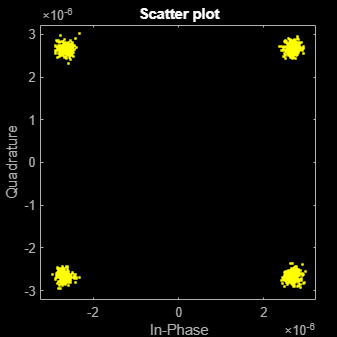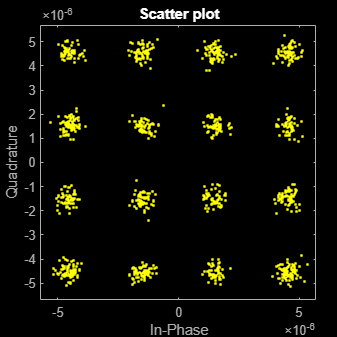# comm.ThermalNoise

## Description

The `comm.ThermalNoise` System object™ object simulates the effects of thermal noise on a complex baseband signal. For more information, see Algorithms.

To add thermal noise to a complex baseband signal:

1. Create the `comm.ThermalNoise` object and set its properties.

2. Call the object with arguments, as if it were a function.

## Creation

### Syntax

``noise = comm.ThermalNoise``
``noise = comm.ThermalNoise(Name=Value)``

### Description

````noise = comm.ThermalNoise` creates a receiver thermal noise System object. This object adds thermal noise to the complex baseband input signal.```

example

````noise = comm.ThermalNoise(Name=Value)` sets properties using one or more name-value arguments. For example, `SampleRate=2` sets the input signal sample rate to 2.```

## Properties

expand all

Unless otherwise indicated, properties are nontunable, which means you cannot change their values after calling the object. Objects lock when you call them, and the `release` function unlocks them.

If a property is tunable, you can change its value at any time.

Method used to set the noise power, specified as ```'Noise temperature'```, `'Noise figure'`, or ```'Noise factor'```.

Receiver noise temperature, specified in kelvins as a nonnegative scalar. Noise temperature is typically used to characterize receivers because the input noise temperature can vary and is often less than 290 K.

Tunable: Yes

#### Dependencies

This property applies when you set `NoiseMethod` to `'Noise temperature'`.

Data Types: `double` | `single`

Noise figure in dB, specified as a nonnegative scalar. Noise figure describes the performance of a receiver and does not include the effect of the antenna. It is defined only for an input noise temperature of 290 K. The noise figure is the dB equivalent of the noise factor.

Tunable: Yes

#### Dependencies

This property applies when you set `NoiseMethod` to `'Noise figure'`.

Data Types: `double` | `single`

Noise factor, specified as a scalar greater than or equal to 1. Noise factor describes the performance of a receiver and does not include the effect of the antenna. It is defined only for an input noise temperature of 290 K. The noise factor is the linear equivalent of the noise figure.

Tunable: Yes

#### Dependencies

This property applies when you set `NoiseMethod` to `'Noise factor'`.

Data Types: `double` | `single`

Reference load in ohms, specified as a positive scalar. The reference load value is used to compute the voltage levels based on the signal and noise power levels.

Tunable: Yes

Data Types: `double` | `single`

Sample rate in Hz, specified as a positive scalar. The object computes the variance of the noise added to the input signal as kT×`SampleRate`×`ReferenceLoad`. The value k is Boltzmann's constant and T is the noise temperature specified explicitly or implicitly via one of the noise methods.

Data Types: `double` | `single`

Option to add 290 K antenna noise to the input signal, specified as a logical `0` (`false`) or `1` (`true`). To add 290 K antenna noise, set this property to `true`. The total noise applied to the input signal is the sum of the circuit noise and the antenna noise.

#### Dependencies

To enable this property, set the `NoiseMethod` property to `'Noise factor'` or `'Noise figure'`.

Data Types: `logical`

## Usage

### Syntax

``outsignal = noise(insignal)``

### Description

````outsignal = noise(insignal)` adds thermal noise to the complex baseband input signal `insignal` and returns the result in `outsignal`.```

### Input Arguments

expand all

Baseband signal, specified as a complex-valued scalar, NS-element column vector, or NS-by-NC matrix. NS is the number of samples and NC is the number of channels.

Data Types: `double` | `single`
Complex Number Support: Yes

### Output Arguments

expand all

Output signal, returned as a scalar, column vector, or matrix of complex values with the same length and data type as the input signal.

Data Types: `double` | `single`
Complex Number Support: Yes

## Object Functions

To use an object function, specify the System object as the first input argument. For example, to release system resources of a System object named `obj`, use this syntax:

`release(obj)`

expand all

 `info` Characteristic information about thermal noise object
 `step` Run System object algorithm `release` Release resources and allow changes to System object property values and input characteristics `reset` Reset internal states of System object

## Examples

collapse all

Create a thermal noise object with a noise temperature of 290 K and a sample rate of 5 MHz.

`thNoise = comm.ThermalNoise('NoiseTemperature',290,'SampleRate',5e6);`

Generate QPSK modulated data with an output power of 20 dBm.

```data = randi([0 3],1000,1); modData = (10^((20-30)/20)) * pskmod(data,4,pi/4);```

Attenuate the signal by the free space path loss assuming a 1000 m link distance and a carrier frequency of 2 GHz.

```d = 1000; % m f = 2e9; % Hz c = 3e8; % m/s fsl = (4*pi*d*f/c)^2; rxData = modData/sqrt(fsl);```

Add thermal noise to the signal. Plot the noisy constellation.

```noisyData = thNoise(rxData); scatterplot(noisyData)```Create a thermal noise object with a 5 dB noise figure and a 10 MHz sample rate. Include 290 K antenna noise.

```thermalNoise = comm.ThermalNoise('NoiseMethod','Noise figure', ... 'NoiseFigure',5, ... 'SampleRate',10e6, ... 'Add290KAntennaNoise',true);```

Generate QPSK modulated data with an output power of 1 W.

```data = randi([0 15],1000,1); modSig = qammod(data,16,'UnitAveragePower',true);```

Attenuate the signal by the free space path loss assuming a 1 km link distance and a 5 GHz carrier frequency.

```d = 1000; % m f = 5e9; % Hz c = 3e8; % m/s fsl = (4*pi*d*f/c)^2; rxSig = modSig/sqrt(fsl);```

Add thermal noise to the signal and plot its constellation.

```noisySig = thermalNoise(rxSig); scatterplot(noisySig)```Estimate the SNR.

```mer = comm.MER; snrEst1 = mer(rxSig,noisySig)```
```snrEst1 = 22.6611 ```

Decrease the noise figure to 0 dB and plot the resultant received signal. The signal is not completely noiseless because antenna noise is included.

```thermalNoise.NoiseFigure = 0; noisySig = thermalNoise(rxSig); scatterplot(noisySig)```Estimate the SNR. The SNR is 5 dB higher than in the first case, which is expected given the 5 dB decrease in the noise figure.

`snrEst2 = mer(rxSig,noisySig)`
```snrEst2 = 27.8658 ```
`snrEst2 - snrEst1`
```ans = 5.2047 ```

Apply thermal noise to a multichannel signal and confirm that each channel has the same noise floor as returned by the `info` object function.

Create a `comm.ThermalNoise` object.

`tn = comm.ThermalNoise`
```tn = comm.ThermalNoise with properties: NoiseMethod: 'Noise temperature' NoiseTemperature: 290 ReferenceLoad: 1 SampleRate: 1 ```

Apply thermal noise to a multichannel signal and compute the signal variance for each channel.

```x = tn(zeros(100000,3,'like',1i)); 10*log10(var(x))```
```ans = 1×3 -203.9973 -203.9684 -203.9802 ```

Use the `info` object function to return the receiver thermal noise floor.

`info(tn)`
```ans = struct with fields: NoiseFloor: -203.9752 ```

## Algorithms

Wireless receiver performance is often expressed as a noise factor or figure. The noise factor, F, is defined as the ratio of the input signal-to-noise ratio, Si/Ni to the output signal-to-noise ratio, So/No, such that

`$F=\frac{{S}_{i}/{N}_{i}}{{S}_{o}/{N}_{o}}\text{\hspace{0.17em}}.$`

Given the receiver gain G and receiver noise power Nckt, the noise factor can be expressed as

`$\begin{array}{c}F=\frac{{S}_{i}/{N}_{i}}{G{S}_{i}/\left({N}_{ckt}+G{N}_{i}\right)}\\ =\frac{{N}_{ckt}+G{N}_{i}}{G{N}_{i}}\text{\hspace{0.17em}}.\end{array}$`

The IEEE® defines the noise factor assuming that noise temperature at the input is T0, where T0 = 290 K. The noise factor is then

`$\begin{array}{c}F=\frac{{N}_{ckt}+G{N}_{i}}{G{N}_{i}}\\ =\frac{GkB{T}_{ckt}+GkB{T}_{0}}{GkB{T}_{0}}\\ =\frac{{T}_{ckt}+{T}_{0}}{{T}_{0}}\text{\hspace{0.17em}}.\end{array}$`

k is Boltzmann's constant. B is the signal bandwidth. Tckt is the equivalent input noise temperature of the receiver and is expressed as

`${T}_{ckt}={T}_{0}\left(F-1\right)\text{\hspace{0.17em}}.$`

The overall noise temperature of an antenna and receiver Tsys is

`${T}_{sys}={T}_{ant}+{T}_{ckt}\text{\hspace{0.17em}},$`

where Tant is the antenna noise temperature.

The noise figure NF is the dB equivalent of the noise factor and can be expressed as

`$NF=10{\mathrm{log}}_{10}\left(F\right)\text{\hspace{0.17em}}.$`

The noise power can be expressed as

`$N=kTB={V}^{2}/R,$`

where V is the noise voltage expressed as

`${V}^{2}=kTBR,$`

and R is the reference load.

## Version History

Introduced in R2012a

expand all